# Sliding motion for adjacent blocks along an inclined plane

This article discusses a scenario/arrangement whose statics/dynamics/kinematics can be understood using the ideas of classical mechanics.
View other mechanics scenariosThe two blocks are adjacent to each other on the incline.

The scenario here is of two adjacent dry cuboidal blocks as shown in the figure, on an incline of a fixed inclined plane making an angle$\theta$ with the horizontal.

The upper mass is denoted as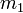$m_1$, and the coefficients of static and kinetic friction between that mass and the incline are denoted$\mu_{s1}$ and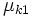$\mu_{k1}$. The lower mass is denoted as$m_2$, and the coefficients of static and kinetic friction between that mass and the incline are denoted$\mu_{s2}$ and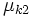$\mu_{k2}$.

The extremes are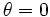$\theta = 0$ (whence, the plane is horizontal) and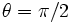$\theta = \pi/2$ (whence, the plane is vertical).

We assume that the block undergoes no rotational motion, i.e., it does not roll or topple. Although the diagram here shows a block with square cross section, this shape assumption is not necessary as long as we assume that the block undergoes no rotational motion.

## Behavior assuming system initially at rest

Note that the answer in the third column must be yes if the first two columns are yes and no if the first two columns are no.

Is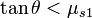$\tan \theta < \mu_{s1}$? Is$\tan \theta < \mu_{s2}$? Is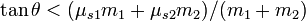$\tan \theta < (\mu_{s1}m_1 + \mu_{s2}m_2)/(m_1+m_2)$? Is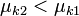$\mu_{k2} < \mu_{k1}$? Behavior Do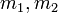$m_1,m_2$ stay together?
Doesn't matter Yes Yes Doesn't matter Both blocks remain stationary. Yes
Yes No Doesn't matter Doesn't matter$m_2$ slides down,$m_1$ stays stationary. No
No No No Yes Both slide down, but$m_2$ slides down faster. No
No No No No Both slide down together, with acceleration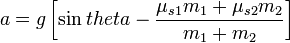$a = g\left[\sin theta - \frac{\mu_{s1}m_1 + \mu_{s2}m_2}{m_1 + m_2}\right]$. Yes